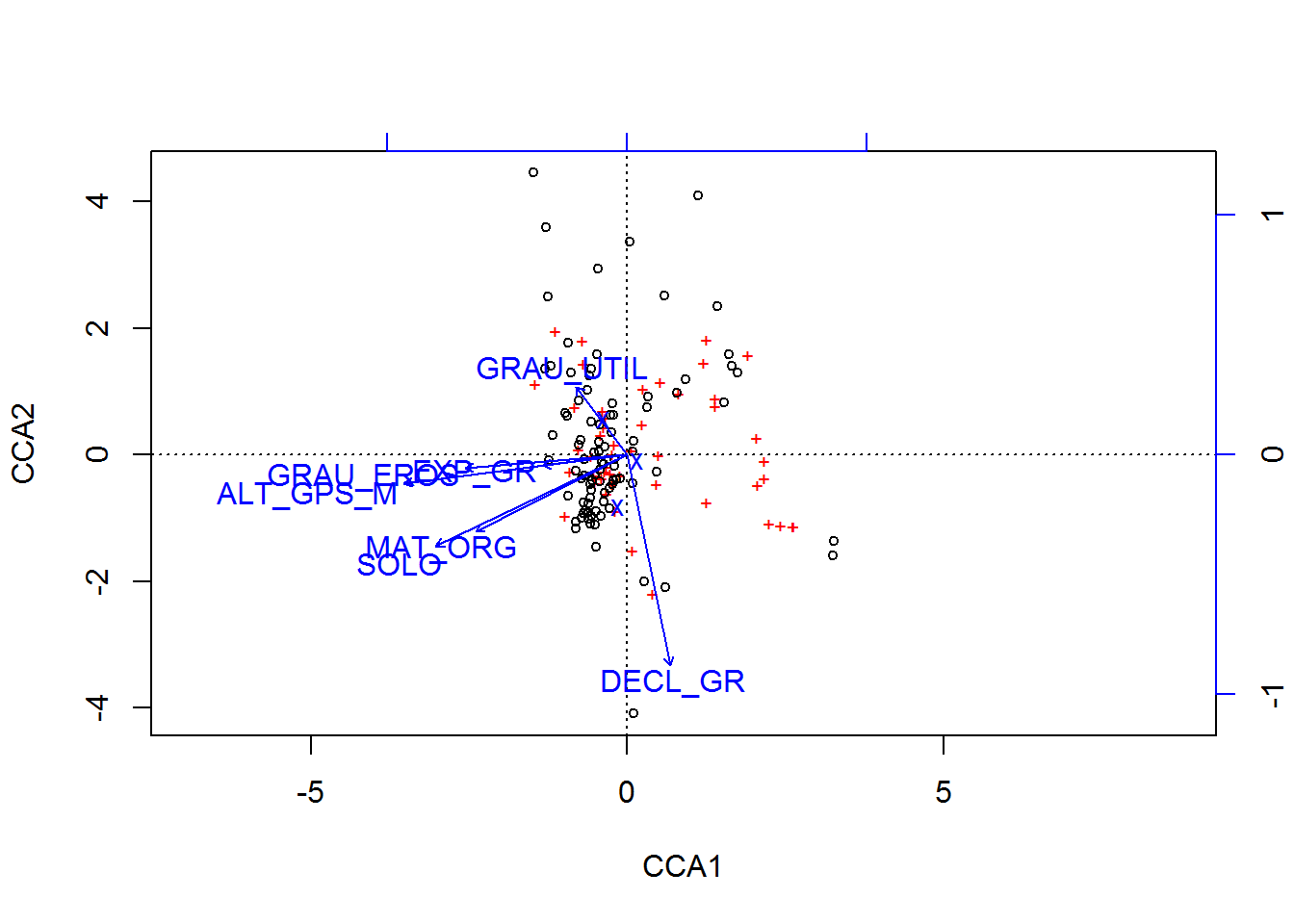#BIS-Fogo

### Site Tools

en:learning:schools:s02:code-examples:sm-ce-03-03

## C01-3: Ordination analysis

The following example uses data from a field survey of areas in the Fogo natural park in 2007 by K. Mauer. For more information, please refer to this report.

Regarding libraries, the following packages are necessary.

library(vegan)
## Loading required package: permute
## This is vegan 2.3-0

#### Multivariate data mining

Ordination analysis is a multivariate data mining method which reduces the dimensionality of an ecological data set and re-arranges the reduced data values onto a two dimensional space in such a way that potential relationship patterns between the individual ecological information becomes as apparent as possible. Therefore, common data reduction and transformation techniques like principal component analysis, multi-dimensional scaling or correspondence analysis is used.

Maybe the most commonly applied technique in ordination analysis is the correspondence analysis. It is heavily used to identify and delineate communities of species (i.e. groups of species which frequently occur together at one location). The correspondence analysis uses a Chi-square distance matrix as basis for its eigenalanysis. Therefore, the observed vs. expected values of each species at each location are computed following the commonly used Chi-square formula:

(observed value - expected value)2 / expected value

In addition, environmental factors can be considered as so called constraining variables.

ordination<-cca(data_species ~ GRAU_EROS + SOLO + CAT_USO + DECL_GR + EXP_GR +
MAT_ORG + ALT_GPS_M + GRAU_UTIL, data = data)
plot(ordination)In order to get an idea on the significance of the constraining variables, a simple analysis of variance (ANOVA) can be calculated using the ordination object.

anova(ordination, by = "terms", permu = 999)
## Permutation test for cca under reduced model
## Terms added sequentially (first to last)
## Permutation: free
## Number of permutations: 999
##
## Model: cca(formula = data_species ~ GRAU_EROS + SOLO + CAT_USO + DECL_GR + EXP_GR + MAT_ORG + ALT_GPS_M + GRAU_UTIL, data = data)
##           Df ChiSquare      F Pr(>F)
## GRAU_EROS  1    0.4003 4.2001  0.001 ***
## SOLO       1    0.2855 2.9961  0.009 **
## CAT_USO    2    0.3567 1.8713  0.006 **
## DECL_GR    1    0.2743 2.8785  0.001 ***
## EXP_GR     1    0.1577 1.6551  0.045 *
## MAT_ORG    1    0.2587 2.7147  0.001 ***
## ALT_GPS_M  1    0.2660 2.7906  0.004 **
## GRAU_UTIL  1    0.1146 1.2029  0.376
## Residual  85    8.1008
## ---
## Signif. codes:  0 '***' 0.001 '**' 0.01 '*' 0.05 '.' 0.1 ' ' 1